Next: Ecliptic Coordinates Up: Spherical Astronomy Previous: Celestial Coordinates

# Ecliptic Circle

During the course of a year, the sun's intrinsic motion causes it to trace out a fixed circle which bisects the celestial sphere. This circle is known as the ecliptic. The sun travels around the ecliptic from west to east (i.e., in the opposite direction to the celestial sphere's diurnal rotation). Moreover, the ecliptic circle is inclined at a fixed angle of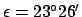to the celestial equator. This angle actually represents the fixed inclination of the earth's axis of rotation to the normal to its orbital plane.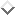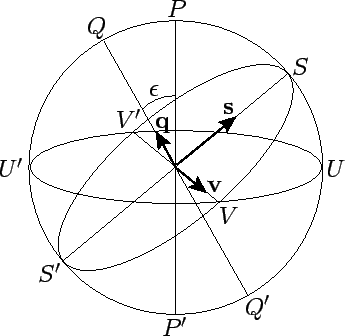The vernal equinox,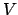, is defined as the point at which the ecliptic crosses the celestial equator from south to north (in the direction of the sun's ecliptic motion)--see Fig. 5. Likewise, the autumnal equinox,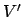, is the point at which the ecliptic crosses the celestial equator from north to south. In addition, the summer solstice,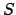, is the point on the ecliptic which is furthest north of the celestial equator, whereas the winter solstice,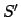, is the point which is furthest south. It follows that the lines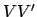and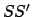are perpendicular. Let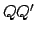be the normal to the plane of the ecliptic which passes through the earth, as shown in Fig. 5. Here,is termed the northern ecliptic pole, and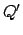the southern ecliptic pole. It is easily demonstrated that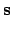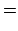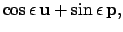(4)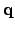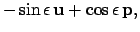(5)

where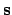is a unit vector which is directed from the earth to the summer solstice, anda unit vector which is directed from the earth to the north ecliptic pole--see Fig. 5. We can also write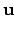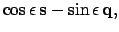(6)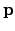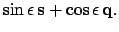(7)

Thus,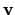,, andconstitute another right-handed, mutually perpendicular, set of unit vectors.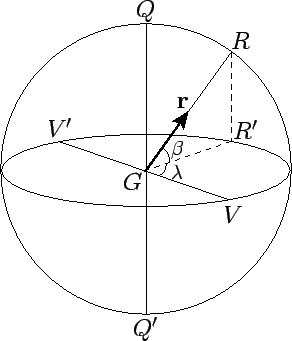Next: Ecliptic Coordinates Up: Spherical Astronomy Previous: Celestial Coordinates
Richard Fitzpatrick 2010-07-21